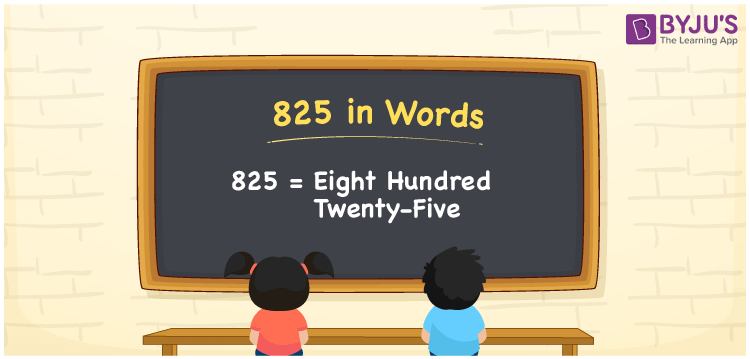# 825 in Words

In the Indian and the International System of numerals, the number 825 in words is Eight Hundred Twenty-five. As we know, the number 825 is a cardinal number, since it helps to express the specific quantity. For the conversion of numbers to words, the place value system proves useful. Now, let us have a look at the procedure of writing the number 825 in words.

 825 in Words: Eight Hundred Twenty-five. Eight Hundred Twenty-five in Numerical Form: 825.

## 825 in English Words## How to Write 825 in Words?

The below table shows the place values of the three-digit number 825:

 Hundreds Tens Ones 8 2 5

The expanded form of 825 is as follows:

= 8 × Hundred + 2 × Ten + 5 × One

= 8 × 100 + 2 × 10 + 5 × 1

= 800 + 20 + 5

= 825

= eight hundred twenty-five

Hence, 825 in words is Eight hundred twenty-five.

The number 825 appears after 824 but before 826.

825 in words – Eight hundred twenty-five

Is 825 an odd number? – Yes

Is 825 an even number? – No

Is 825 a perfect square number? – No

Is 825 a perfect cube number? – No

Is 825 a prime number? – No

Is 825 a composite number? – Yes

## Frequently Asked Questions on 825 in Words

Q1

### How to spell 825 in English words?

825 in words is eight hundred twenty-five.

Q2

### Simplify 800 + 25, and express it in words.

Simplifying 800 + 25, we get 825. Hence, 825 in words is eight hundred twenty-five.

Q3

### Is 825 a prime number?

No, 825 is not a prime number.